# Defensor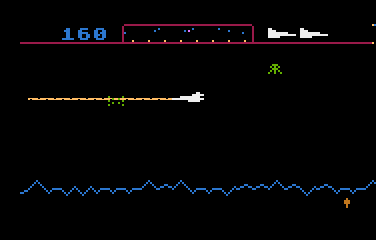This is a little game for the 2018 NOMAM's BASIC 10-liners Contest. This program fits in the EXTREM-256 category, and it was written using FastBasic 3.4 for the 8-bits ATARI XL/XE. Development started on 2018-02-27, and it took 3+3+10 days. The final version's date is 2018-03-23.

UPDATE: It obtained the 1st place of 17 entries in the category.

## Description

Protect the humanoids from the evil aliens and rescue those who have been abducted.

## Instructions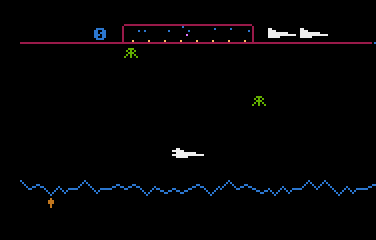You are the captain of a space ship that must protect the humanoids in the landscape from alien abduction. The scanner (radar) in the top of the screen will help you to find aliens and to identify abducted humanoids.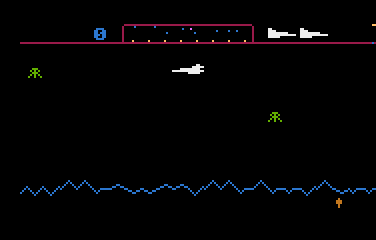The ship is always moving to the left or to the right of the screen, and you can change the direction and/or accelerate moving the joystick to the required direction.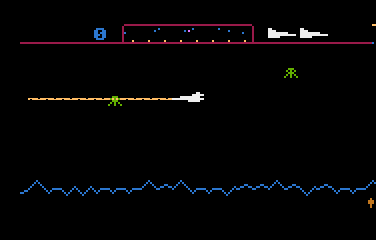You can fire to destroy the aliens before they reach the humanoids only if they are at a considerable height. You cannot fire if you are near the surface.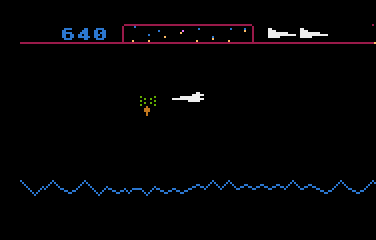Abducted humanoids can be saved before the alien reaches the top of the playfield if you shoot the alien and pick the humanoid before he dies at the end of a free fall. Be careful: if you hit the humanoid, he'll die.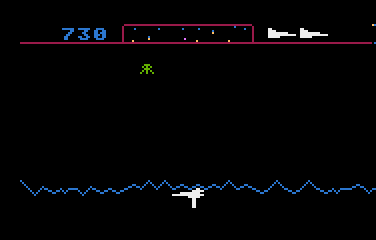While you are carrying an humanoid, you must perform a flush flight on the surface and the humanoid will descend when he finds a safe point. Note that when you are carrying the humanoid, both are exposed to an alien attack. You can neither fire to more aliens nor catch another falling humanoid.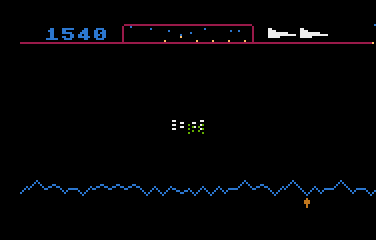Watch out! When you kill an alien, you cannot pass between its remains. You must change the flying direction or move up or down to avoid them.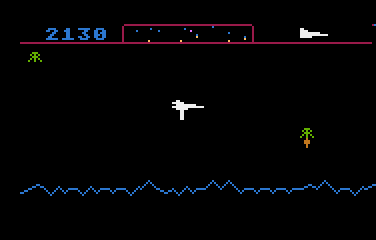When you kill an alien, you will earn 20 points for every living humanoid, i.e. 160 points if all the humanoids are alive, but just 20 points if there is only one. Then, you must protect all the humanoids to quickly increase your score. Hint: You will earn 90 extra points when you pick a falling humanoid and 200 points more when the humanoid drops off on the surface.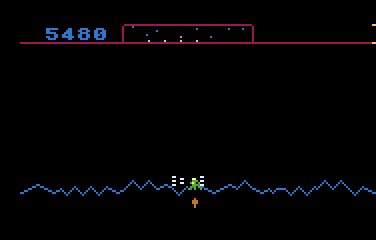You only have 3 space ships to protect the humanoids. The game is over when all of them crached into an alien or its remains, or when all the humanoids have been abducted.

Get the DEFENSOR.ATR file and set it as drive 1 in a real Atari (or emulator). Turn on the computer and the game should start after loading.

## The code

The abbreviated BASIC code is the following. Please note that FastBasic does not use line numbers!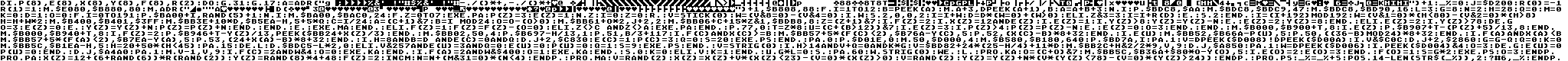The full and expanded BASIC listing is:

 ```dim p(8),e(8),x(8),y(8),f(8),r(2) ``` Declares the arrays: P(): height of each humanoid. There are 8 humanoids distributed in 8 similar zones. E(): status of each humanoid: 0=dead 1=alive 2=captured 3=falling X(): horizontal coordinate of an alien in its zone, from 0 to 23. Y(): vertical coordinate of an alien in double scan lines. F(): status of each alien: 0=dead 1=exploded 2=alive R(): Just a sign for direction: 0 is -1 (left) and 1 is 1 (right) ```do ``` Game loop ``` graphics 31 ``` Cleans 8K of upper RAM. ``` graphics 17 ``` Sets graphics mode 1 (ANTIC 6), color text mode with a size of 40x24. ``` a=adr("{data}")+1 ``` Lots of packed data to be stored in many places, including hardware registers. ``` _%=0 ``` This is the only floating point variable of the program. It stores the score. ``` j=\$D200 ``` Defines a constant for the memory address of sound channels. Sometimes is shorter to use a POKE instead of a SOUND statement. ``` r(0)=-1 r(1)=1 ``` ``` move \$E080,\$B880,80 move adr("{data}")+1,\$B808,88 ``` Defines a charset: some chars are the digits (copied from ROM) and some are from the embeded data. ``` for i=1 to 12 b=peek(a) move a+3,dpeek(a+1),b a=a+b+3 next i ``` Loads the configuration from the packed data. This includes the scanner buffers, bits position table for the scanner, the laser pattern, the bitmaps for the P/M, the explosion sound sequence, the color palette, P/M setup, Display List modification, screen initialization and charset selection. ``` poke \$BDC8,\$AA move \$BDC8,\$BDC9,47 move \$BDC8,\$BD90,16 ``` Draw horizontal lines at the top. ``` l=3 g=8 n=2 h=28 q=0 m=0 k=0 d=1 o=0 ``` Initializes game variables. ``` for i=0 to 191 poke \$BA00+i,rand(5)+1 next i move \$BA00,\$BAC0,24 ``` Creates the surface. ``` for z=0 to 7 exec pa p(z)=3 e(z)=1 next z ``` Places humanoids and aliens in the playfield. ``` i=0 z=0 ``` ``` repeat ``` Game loop ``` v=stick(0) w=(v&8=0)-(v&4=0) ``` Checks if the joystick was moved horizontally. ``` if w sound 2,0,0,2 i=i+w d=d*(w=0)+(w>0) ``` If it was moved horizontally, changes the direction or accelerates if it is currently going in that direction. ``` elif z&3=3 i=i+r(d) ``` If it was NOT moved horizontally, keeps the current direction, but advances slowly (one of every 4 steps) ``` else sound 2 endif ``` Turns off the propulsion sound if the joystick was not moved horizontally. ``` i=(i+192) mod 192 ``` Adjusts the current position of the map. ``` w=(v&1=0)*(h<80)-(v&2=0)*(h>8) h=h+w*2 ``` Checks for a vertical movement of the joystick. ``` move \$B400,\$B401,\$3FF ``` Cleans the P/M area. ``` move \$BB3E+10*d,\$B5EA-h,5+5*q ``` Adds the battleship to the buffer. ``` c=i/24 a=(c+1)&7 b=i mod 24 ``` Calculates displayable section of the playfield. C is left zone and A is right zone. B is the begining horizontal position of the left zone. ``` o=o-(o>0) move \$BB61+o*2,j+2,2 ``` If there was an explosion, plays a sound. ``` move \$BB06+c+15*z&1,\$BDB8,8 ``` Updates the scanner. ``` z=(z+1)&7 ``` Updates one alien at a time. This counter determines which one. ``` if f(z)=2 ``` Alien is alive? ``` if x(z)=12 and e(z) ``` Is the alien just over an alive humanoid? ``` if e(z)=1 ``` Is the humanoid in the surface? ``` if y(z)>8 y(z)=y(z)-n ``` Descend a bit to abduct the humanoid if already too high... ``` else e(z)=2 y(z)=8 endif ``` ... or pick the humanoid. ``` elif e(z)=2 ``` Is the humanoid already abducted? ``` if y(z)>78 dec g o=3 e(z)=0 ``` Kill the humanoid if the alien reached the top of the screen... ``` else y(z)=y(z)+n p(z)=p(z)+n endif endif ``` ... or ascend a bit wit the abducted humanoid. ``` else exec ma endif ``` Moves the alien. ``` elif f(z)=1 f(z)=f(z)-(o=0) ``` If the alien was just killed, keep its remains for a while. ``` elif z<>c and z<>a exec pa endif ``` Puts a new alien in the zone only if that zone is not being displayed. ``` poke 77,0 ``` Resets attract counter. ``` t=z*8 ``` ``` u=(c+(b>12))&7 ``` Computes which of the zones to be displayed on next frame refresh has the humanoid that would be displayed. ``` if e(z)=3 if p(z)>4 p(z)=p(z)-n else e(z)=0 o=3 endif endif ``` If the humanoid is falling, move him down a bit or kill him if he reached the surface. ``` move \$B000,\$B900+t,8 if e(z) poke \$B907+t-(p(z)+5)/13,8 endif ``` Updates the humanoid position in the scanner. ``` move \$B000,\$B940+t,8 if f(z)=2 poke \$B946+t-y(z)/13,peek(\$BB24+x(z)/3) endif ``` Updates the alien position in the scanner. ``` move \$BB02,50,4 ``` Resets the horizontal position buffer for Players, moving all out of the screen. ``` poke \$B697-h/13,1 poke 51,b/3+117 ``` Updates the ship in scanner. ``` if f(c) and x(c)>=b move \$BB57+5*(f(c)<2),\$B76A-y(c),5 poke 52,(x(c)-b)*8+32 endif ``` Places the alien from the zone at the left in screen buffer if it should be displayed. ``` if e(u) move \$BB52,\$B66A-p(u),5 poke 50,((36-b) mod 24)*8+32 endif ``` Places the humanoid in the screen buffer if it should be displayed. ``` if f(a) and x(a)14 and v+q=0 and k*g ``` Checks if it is able to fire: enough height, not carrying a rescued humanoid, not killed in a crash and living humanoids left. ``` v=\$BD82+24*(25-h/4)+11*d move \$BB2C+h&2/2*9,v,9 ``` Computes where should the laser been drawed. The laser is displayed using modified ANTIC 6 fonts. ``` dpoke j,\$A850 ``` Shorter and faster than an abbreviated SOUND 0,80,10,8 statement. ``` pause 1 w=dpeek(\$D006) ``` Waits for a frame refresh to detect laser collisons with aliens (registers for P2 and P3). ``` if peek(\$D004)&4 o=3 dec g e(u)=0 p(u)=0 endif ``` If an abducted humanoid was hit, remove it from the game. ``` dpoke j,\$A4A0 ``` Shorter and faster than abbreviated SOUND 0,160,10,4 statement. ``` pause 1 move v-1,v,9 ``` Removes the laser shot from the screen. ``` if f(c)=2 and w&4 o=0 exec ka endif ``` Left alien was hit? ``` if f(a)=2 and w&\$400 o=1 exec ka endif ``` Right alien was hit? ``` sound 0 k=0 ``` Turns off the firing sound and disables the trigger. ``` elif v k=1 endif ``` Reenables the trigger if it was released. There is no continuous fire in this game! ``` until g*l=0 ``` The game loop continues until there are no more ships or no more humanoids. ``` sound ``` Turns off all remaining sounds. ``` pause 60 while strig(0) wend ``` Waits for the trigger to start a new game. ```loop ``` Repeats the main loop. ```proc ka o=(c+o)&7 move \$BB5C,\$B36A+\$80*o-y(o),5 if e(o)=2 e(o)=3 endif f(o)=1 s=g*2 exec ps o=3 endproc ``` Subroutine to kill left (O=0) or right(o=1) alien. If it had an abducted humanoid, releases it in a free-fall state. ```proc pa x(z)=12+(6+rand(6))*r(rand(2)) y(z)=rand(8)*4+48 f(z)=2 inc m n=n+(m&31=0)*(n<4) endproc ``` Subroutine to add an alien in the selected Z zone. The vertical movement delta for the aliens and free-fall of humanoid is increased every 32 new aliens to increase game difficulty. ```proc ma v=rand(2) x(z)=x(z)+v*(x(z)<23)-(v=0)*(x(z)>0) v=rand(2) y(z)=y(z)+n*(v*(y(z)<78)-(v=0)*(y(z)>24)) endproc ``` Moves the alien randomly inside the bounds of the selected Z zone. ```proc ps _%=_%+s position 14-len(str\$(_%)),2 ? #6,_% endproc ``` Subroutine to increase the score in the S specified points.